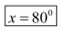• +91 9971497814
• info@interviewmaterial.com

# RD Chapter 16- Circles Ex-16.4 Interview Questions Answers

### Related Subjects

Question 1 : In the given figure, O is the centre of the circle. If ∠APB∠APB= 50°, find ∠AOB and ∠OAB.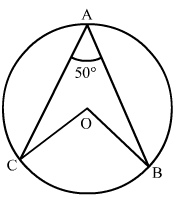APB = 500 (Given)

By degree measure theorem: AOB = 2APB

AOB = 2× 500 = 1000

Again, OA = OB [Radius of circle]

Then OAB = OBA [Angles opposite to equal sides]

Let OAB = m

In ΔOAB,

By angle sum property: OAB+OBA+AOB=1800

=> m + m + 1000 =1800

=>2m = 1800 –1000 = 800

=>m = 800/2 = 400

OAB = OBA = 400

Question 2 : In the given figure, it is given that O is the centre of the circle and ∠AOC = 150°. Find ∠ABC.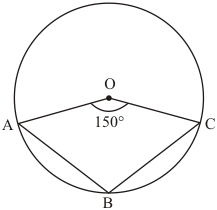It is given that O is the centre of circle and A, B and C are points on circumference.
(Given)We have to find ∠ABC
The angle subtended by an arc of a circle at the centre is double the angle subtended by it at any point on the remaining part of the circle.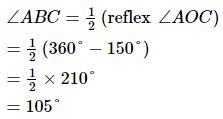Hence,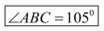Question 3 : In the given figure, O is the centre of the circle. Find ∠BAC.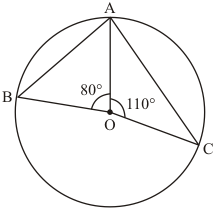It is given that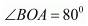And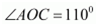(given)We have to find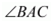In given triangle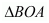(Given)
OB = OA               (Radii of the same circle)
Therefore,   is an isosceles triangle.
So, ∠OBA=∠OAB      ∠OBA=∠OAB                ..… (1)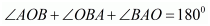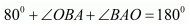(Given)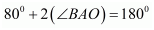[From (1)]
So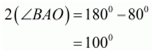Again from figure,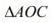is given triangle and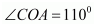Now in,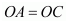(Radii of the same circle)

∠OAC=∠OCA    ∠OAC=∠OCA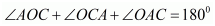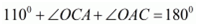(Given that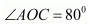)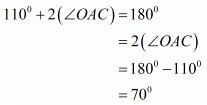Then,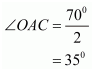Since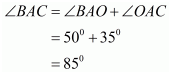Hence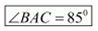Question 4 :
If O is the centre of the circle, find the value of x in each of the following figures.
(i)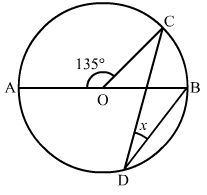(ii)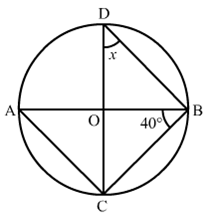(iii)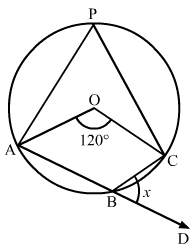(iv)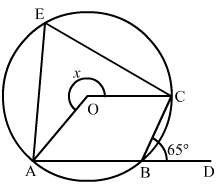(v)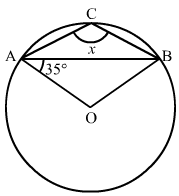(vi)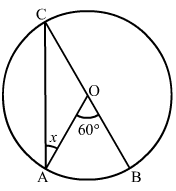(vii)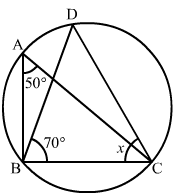(viii)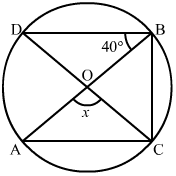(ix)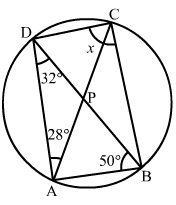(x)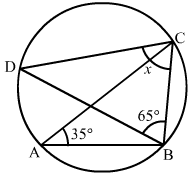(xi)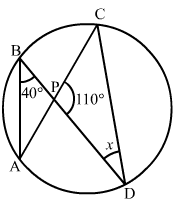(xii)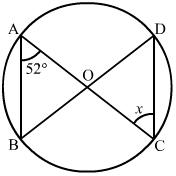We have to find   in each figure.
(i) It is given that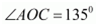∠AOC+∠COB=180°   [Linear pair]∠AOC+∠COB=180°   Linear pair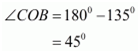As we know the angle subtended by an arc of a circle at the centre is double the angle subtended by it at any point on the remaining part of the circle.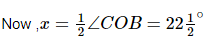Hence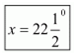(ii) As we know that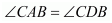= x                 [Angles in the same segment]
line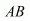is diameter passing through centre,
So,

∠BCA= 90°       [Angle inscribed in a semicircle is a right angle ]∠BCA= 90°       Angle inscribed in a semicircle is a right angle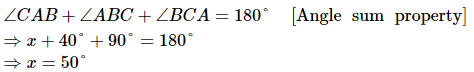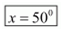(iii) It is given that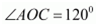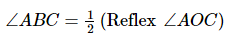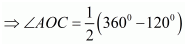SoAnd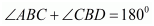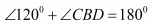Then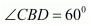Hence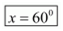(iv)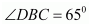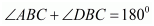(Linear pair)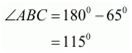And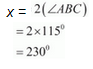Hence,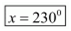(v) It is given that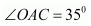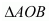is an isosceles triangle.Therefore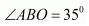And,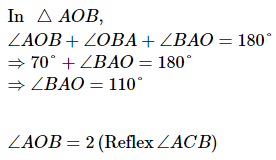Hence,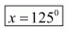(vi) It is given that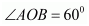And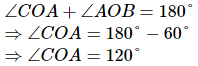ΔOCA is an isosceles triangle.∆OCA is an isosceles triangle.
So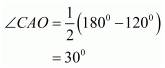Hence,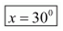(vii)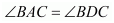(Angle in the same segment)In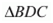we have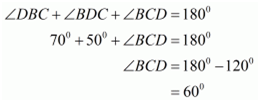Hence(viii)As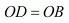(Radius of circle)

Therefore,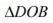is an isosceles triangle.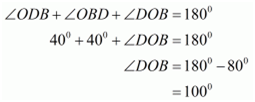So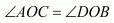(Vertically opposite angles)
Hence,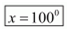(ix) It is given that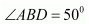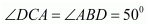…… (1)          (Angle in the same segment)
Because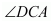and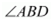are on the same segment   of the circle.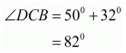Hence,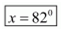(x) It is given that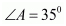Hence,